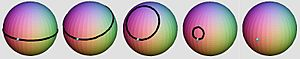# Poincaré conjecture facts for kids

Kids Encyclopedia Facts
FieldA compact 2-dimensional surface without boundary is topologically homeomorphic to a 2-sphere if every loop can be continuously tightened to a point. The Poincaré conjecture asserts that the same is true for 3-dimensional spaces. Geometric topology Henri Poincaré 1904 Grigori Perelman 2006 Geometrization conjecture Zeeman conjecture Spherical space form conjecture Thurston elliptization conjecture Generalized Poincaré conjecture

The Poincaré Conjecture is a question about spheres in mathematics. It is named after Henri Poincaré, the French mathematician and physicist who formulated it in 1904.

The sphere (also called the 2-sphere, as it is a 2-dimensional surface, although it is usually seen as inside a three dimensional space) has the property that any loop on it can be contracted to a point (if a rubber band is wrapped around the sphere, it is possible to slide it down to a point). Mathematicians say that the 2-sphere is simply connected. Other spaces do not have this property, for example the donut: a rubber band that goes around the whole donut once cannot be slid down to a point without it leaving the surface.

Mathematicians knew that this property was unique to the 2-sphere, in the sense that any other simply connected space that does not have edges and is small enough (in mathematician terms, that is compact) is in fact the 2-sphere. It is no longer true if we remove the idea of smallness however, as an infinitely large plane is also simply connected. Also, a regular disk (a circle and its interior) is simply connected, but it has an edge (the bounding circle).

The conjecture asks whether the same is true for the 3-sphere, which is an object living naturally in four dimensions. This question motivated much of modern mathematics, especially in the field of topology. The question was finally settled in 2002 by Grigori Perelman, a Russian mathematician, with methods from geometry, showing that it is indeed true. He was awarded a Fields medal for his work, which he declined.

The Poincaré conjecture can also be extended to higher dimensions: this is the generalised Poincaré conjecture. Surprisingly, it was easier to prove the fact for higher-dimensional spheres: In 1960, Smale proved it to be true for the 5-sphere, 6-sphere and higher, and in 1982, Freedman proved that it was also true for the 4-sphere, for which he was awarded a Fields medal.

## Images for kidsPoincaré conjecture Facts for Kids. Kiddle Encyclopedia.